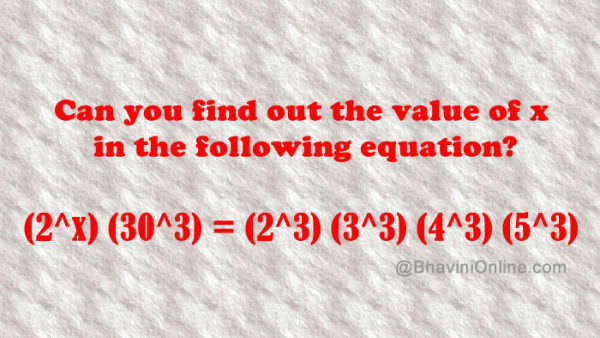# Find Value of x in (2^x) (30^3) = (2^3) (3^3) (4^3) (5^3)

Have fun with numbers with the help of this riddle.

Can you find out the value of x in the following equation?

## (2x) (303) = (23) (33) (43) (53)

This one is for all the maths fans who like to play with the numbers.So were you able to solve the riddle? Leave your answers in the comment section below.